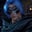RELATED TAGS

sed
communitycreator

# What are Sed's special characters?Gutha Vamsi Krishna

Sed is the Stream Editor, which is useful while working with text files.

In this shot, we will learn about the special characters in Sed.

There are two special characters in SED:

1. The = command
2. The & command

We will discuss these special characters in the rest of the shot.

### = command

The = command writes the line numbers to the output stream followed by its content.

Let’s look at an example to understand it better.

### Example

We use data.txt as the input file in the following example. This has nine lines of content.

We use the sed command and provide the = command followed by the file name.

If we run the code snippet below, it will print the line number followed by its content.

main.sh
data.txt
sed '=' data.txt

We can restrict to only certain content. This means the command will print the line numbers to certain content. For the rest of the content, it doesn’t print any lines.

We can achieve this by providing the line range as follows. Here, we provide the range as 1,4. Hence, it will print the line numbers until 4.

main.sh
data.txt
sed '1,4 =' data.txt

We can also count the lines by providing ＄. This only prints the line number for the last line. It also prints the rest of the content present in the pattern buffer. We can suppress this default behavior using -n.

main.sh
data.txt
sed -n '\$ =' data.txt

### & command

The & command stores the pattern when the pattern is matched.

Let’s go through an example to understand it better.

### Example

First, go through the content of the data.txt file given below.

As we can see, every line starts with a line number associated with it. If we want to insert a term before every line number, we use the sed command to replace the content where the line number is present.

In the code snippet below, we use a regular expression, [[:digit:]], to find the digits. We replace these digits with the The  string. We pass data.txt as an input stream to the sed command.

main.sh
data.txt
sed 's/[[:digit:]]/The /' data.txt

When we run the code snippet above, we lose the line number while inserting the The keyword before every line. We can solve this using the & operator because it stores the pattern. This means that it stores every line number in this case.

main.sh
data.txt
sed 's/[[:digit:]]/The &/' data.txt

RELATED TAGS

sed
communitycreator

CONTRIBUTORGutha Vamsi Krishna
RELATED COURSES

View all Courses

Keep Exploring

Learn in-demand tech skills in half the time